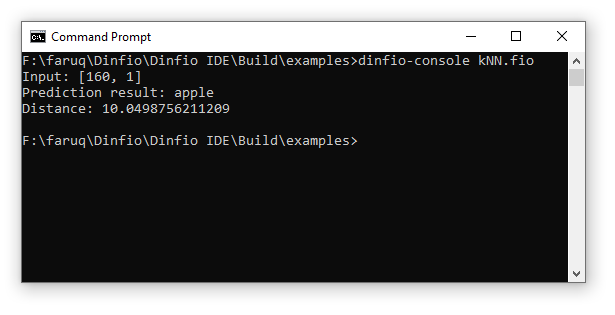k-Nearest Neighbours in Dinfio - Muhammad Faruq NuruddinsyahAlgorithm & Code Sharing   ⋅   Writings   ⋅   Arts   ⋅   About k-Nearest Neighbours in Dinfio This is the implementation of simple k-Nearest Neighbours (kNN) classifier in Dinfio Language with Euclidean distance as the distance metric.Screenshot:Source Code: ```' ------------------------------------ ' Machine Learning Example in Dinfio ' ------------------------------------ ' k-Nearest Neighbours classifier ' By: Faruq ' ------------------------------------   import math   start var KNN: knn = KNN(3)   training_features = [[140, 1], [130, 1], [150, 0], [170, 0]] training_labels = ["orange", "orange", "apple", "apple"] testing = [160, 1]   knn.train(training_features, training_labels) prediction = knn.predict(testing)   writeln("Input: [" & testing & ", " & testing & "]") writeln("Prediction result: " & prediction.label) writeln("Distance: " & prediction.distance) stop   class KNN field x_train, y_train field k   function construct(k) this.k = k stop   function train(features, labels) this.x_train = features this.y_train = labels stop   function predict(test) best_distance = this.distance(this.x_train, test) prediction = this.y_train result = object()   for i, 1, size(this.x_train) - 1 dist = this.distance(this.x_train[i], test)   if dist < best_distance best_distance = dist prediction = this.y_train[i] endif endfor   result.label = prediction result.distance = best_distance   return result stop   function distance(point_1, point_2) sum = 0   for i, 0, size(point_1) - 1 sum += (point_1[i] - point_2[i]) ^ 2 endfor   return sqrt(sum) stop endclass``` Lihat semua daftar ACS - Download: knn.fio - Tanggal: 10 Agustus 2020 - Kategori: Dinfio © 2021 Muhammad Faruq Nuruddinsyah. All rights reserved.